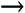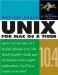# Using Variables

Using Variables

Can you think back on all that stuff you learned in high school algebra? Things like

a 2 b 2 = ( a b )*( a + b )

or

E = MC 2

Those letters are variables, essentially storage containers with names . Any time you see a variable, you are supposed to replace it with whatever is in the container. That's really all a "variable" is.

For example, if we take the first formula above, and store 5 in the variable a and 3 in the variable b , then when we access a we get 5 and when we access b we get 3; so we would read the formula as

5 2 3 2 = (5 3)*(5 + 3)

(which, if you are curious , works out to 25 9 = ( 2 )*(8), which is 16 = 16).

It is common in programming to want to use the contents of a variable inside a string of text; for example, if you have

"Hello \$ name "

you probably want to replace the variable \$name with its contents to create a new string of text. In computer programming, the act of replacing a variable with its contents to create a new string of text is called interpolating (which is not at all the same as turning someone over to the international police organization Interpol).

All computer programming makes heavy use of variables. There are two major things you do with variables: assign something to them (store information in the container) and interpolate themthat is, replace the variable(s) with the contents of the container(s).

In a shell script, you assign a value to a variable using the = operator. It copies the value on the right side into the variable on the left side. For example:

greeting="Top of the morning to you."

or

maximum_length=25

or

` # Speed of light in furlongs/fortnight C=1802617500000 `

Variables are replaced with their contents (that is, interpolated) any time they appear in a command line with a \$ in front of their name:

echo "Then he said: \$greeting"

The following task creates a script that assigns a value to a variable and then interpolates it in a command line. This is commonly done to store an error or warning message in a variable so that it may be used in several places in the script. That way, if you want to change the message, you only have to change it in one place.

To assign a value to a variable and then interpolate it:

1.
Edit a new script file. For example,

vi newscript.sh

2.
The first line is the same as before:

#!/bin/sh

3.
Enter some comments to explain what the script does:

` # This script assigns values tovariables # and then interpolates them. `

4.
line1="The queen , my lord, is dead."

5.
line2="She should have diedhereafter."

Assigning a value to a variable in a Bourne shell script is very simple. You start the line with the variable name. This can be any combination of letters, numbers , and the underscore character, _ (for example, a variable named item_23_01 is fine). However, variable names may not begin with a number.

Right after the variable name you put an equals sign ( = ). Do not put any spaces before or after the = sign.

After the = sign comes the value that is stored in the variable. The value can be a number or text. If the value has spaces inside it, enclose the value in quotes.

6.

# Now interpolate the variables.

7.
echo "SEYTON: \$line1"

8.
echo "MACBETH: \$line2"

Interpolating a variable is simply a matter of using the variable name in a command line with \$ added to the beginning of the variable name.

When the shell reads any command line, either one entered at a shell prompt or one found in a script, the first thing the shell does is interpolate variables that start with \$ .

Figure 9.3 is a code listing of a script that assigns a value to a variable and then interpolates it, and Figure 9.4 shows the result of running the script.

##### Figure 9.3. Code listing of a script that assigns values to two variables and then interpolates them.
` #!/bin/sh # This script assigns values to variables # and then interpolates them. line1="The queen, my lord, is dead." line2="She should have died hereafter." # Now interpolate the variables. echo "SEYTON: \$seyton" echo "MACBETH: \$macbeth" `

##### Figure 9.4. Running the script that uses variables.
` localhost:~ vanilla\$  ./myscript.sh  SEYTON: The queen, my lord, is dead. MACBETH: She should have died hereafter. localhost:~ vanilla `

The first one we listed shows how to calculate the difference between two squared numbers. So the difference between 9 2 and 4 2 is (9 4) times (9 + 4). That is, 81 16 equals 5 times 13. Check it out. Works every time. Pretty cool, huh? For more, see "The Difference of 2 Squares" (www.mste.uiuc.edu/users/dildine/ sketches /Diff2sq.htm).

The second equation is Einstein 's famous formula expression of his special theory of relativity , that energy ( E ) is equal to mass ( M ) multiplied by the square of the velocity of light ( C ). That formula plus a lot of technology can get you global thermonuclear war if you are not careful. For more, see "Einstein Explains the Equivalence of Energy and Matter" (www.aip.org/history/einstein/voice1.htm).Tip

• If you want to include quotes in the value, then you escape the quotes with backslashes:

response="MACBETH: \"Liar and slave!\""

It is quite common to use the value of a variable inside the assignment of another variable. This is easy to do because the shell performs variable interpolation on all the variables in a line before executing the command at the start of a line.

To use a variable inside another variable:

• Simply use a variable with the \$ inside the value of a new variable.

For example,

` first_name="Alexis" last_name="Pushkin" full_name="\$first_name \$last_name" `

Figure 9.5 is a code listing of a complete script that uses a variable inside the value assigned to another. Figure 9.6 shows the output of the script.

##### Figure 9.5. Code listing of a script that uses variables inside the values assigned to other variables.
` #!/bin/sh # Example of using a variable inside the value # assigned to another variable. directory="/Applications (Mac OS 9)" message="OS 9 applications are in \"\$directory\"" directory="/Applications" echo "\$message" message="OS X applications are in \"\$directory\"" echo "\$message" `

##### Figure 9.6. Output of the script in Figure 9.5.
` localhost:~ vanilla\$  ./newscript.sh  OS 9 applications are in "/Applications (Mac OS 9)" OS X applications are in "/Applications" localhost:~ vanilla `

The first line of output comes from line 8 of the script. Notice how the contents of \$message are what were assigned to it on line 6. The assignment of a new value to \$directory on line 7 does not change what was already assigned to \$message . On line 9, there is a new assignment using \$directory , which has a new value. The second line of output (which comes from line 10 of the script) shows this.

#### Using a command inside a variable

It is very common in shell scripts to store the name of a command in a variable and then use the variable in a command line later in the script. This is commonly done to allow the same command to be used in many places in the script, but to have only one place where it needs to be changed if you decide to use a different command later.

To use a command inside a variable:

1.
Assign the command name to a variable.

For example,

command="ls"

2.
Use the variable (with \$ ) anywhere you want to use the command.

For example,

\$command /usr

Figure 9.7 is a code listing of a script that uses a command in a variable, and Figure 9.8 shows the output of the script.

##### Figure 9.7. Code listing of a script that uses a command name in a variable.
` #!/bin/sh # This script uses a command name in a variable # The du command shows disk usage command="du" # The -s option means "summary". The -k means "in kilobytes" options="-sk" directory="/Developer/ADC Reference Library" # Note the use of quotes around \$directory in the command line echo "Trying \$command with options \$options on '\$directory/*'" \$command \$options "\$directory"/* `

##### Figure 9.8. Output from the script in Figure 9.7.
` localhost:~ vanilla\$  ./diskuse.sh  Trying du with options -sk on '/Developer/ADC Reference Library/*' 672    /Developer/ADC Reference Library/docSet.xml 552852 /Developer/ADC Reference Library/documentation 4 /Developer/ADC Reference Library/index.html 67944  /Developer/ADC Reference Library/indexes 107800 /Developer/ADC Reference Library/referencelibrary 10780  /Developer/ADC Reference Library/releasenotes 23936  /Developer/ADC Reference Library/samplecode 11452  /Developer/ADC Reference Library/technicalnotes 28832  /Developer/ADC Reference Library/technicalqas localhost:~ vanilla `Tips

• Use variables in scripts whenever you want the same thing to appear in more than one place. This minimizes the number of places where you need to make changes.

• Try creating the script in Figure 9.7 and changing the command to ls and options to different options for the ls commandfor example, -ld and/or -F .

#### Using environment variables in a script

In previous chapters ( especially Chapter 7), you learned about a special kind of variable called an environment variable . When you execute a script, you are creating a child process of your shell, and so the script's processes inherit your shell's environment. As a result, you can use all of your shell's environment variables in your scripts. Note that assigning anything into an environment variable in a script does not change the contents of that variable for the parent process (your shell), but sets it for any child processes the script creates.

To use an environment variable in a script:

• Use the environment variable as you would any other variable.

For example,

` echo "Hello \$USER" echo "Your home directory is \$HOME" `

Figure 9.9 is a code listing of a script that uses environment variables, and Figure 9.10 shows the output from the script. Note that the script uses the sudo command (which we introduced in Chapter 8) to gain extra privileges. These privileges are needed because Mac OS X (starting in version 10.4) puts some files in each user's home directory that are not owned by the user. Without them, the du command in the script would fail when it tried to read those files. (The sudo command will be covered in more detail in Chapter 11).

##### Figure 9.9. Code listing of a script that uses environment variables.
` #!/bin/sh # This script uses environment variables command="du" options="-sk" command_line="\$command \$options \$HOME" echo "User is:                 \$USER" echo "Home directory is:       \$HOME" echo "Command line will be:    \$command_line" echo "Disk space usage in kilobytes:" sudo \$command_line `

##### Figure 9.10. Output from the script in Figure 9.9.
` localhost:~ vanilla\$  ./envscript.sh  User is:                vanilla Home directory is:      /Users/vanilla Command line will be:   du -sk /Users/vanilla Disk space usage in kilobytes: Password: 2160    /Users/vanilla localhost:~ vanilla `Unix for Mac OS X 10.4 Tiger: Visual QuickPro Guide (2nd Edition)
ISBN: 0321246683
EAN: 2147483647
Year: 2004
Pages: 161
Authors: Matisse Enzer

Similar book on Amazon